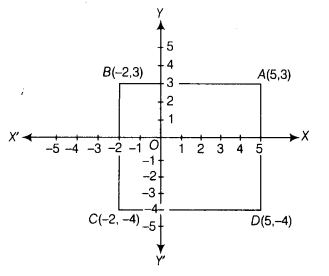# Points A(5,3), B(-2,3) and D(5,-4) are three vertices of a square ABCD

Points A(5,3), B(-2,3) and D(5,-4) are three vertices of a square ABCD. Plot these points on a graph paper and hence, find the coordinates of the vertex C.

The graph obtained by plotting the points A,B and D is given below.To find point C, draw a line parallel to AD and passing through B. Also, draw a line parallel to AB and passing through D.
Thus, the point of intersection of lines drawn above isC.
Clearly, the coordinates of the vertex C are (-2, -4).